Next: Diagonal Gaussian ensemble Up: Ensemble Learning for Independent Previous: Model selection

# Model for the measurements

The measurements vectors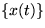are assumed to be generated by a linear mapping A from mutually independent source signals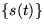and additive Gaussian noise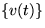.

x(t) = As(t) + v(t)

The components vi(t) of the noise are assumed to have means bi and variances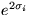. Another way to put this is to say that x(t) has Gaussian distribution with mean As(t)+b and diagonal covariance with components. Each component Aij of the linear mapping is assumed to have zero mean and unit variance.

The distribution of each source signal is a mixture of Gaussians (MOG).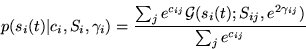The parameters cij are the logarithms of mixture coefficients, S the means and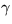the logarithms of the standard deviations of the Gaussians(here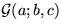denotes a Gaussian distribution over a with mean b and variance c).

The distributions of parameters cij, Sij,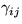,bi andare,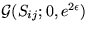,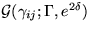,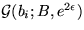and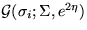.

The prior distribution of the hyperparameters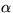,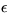,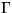,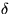, B,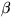,and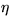is assumed to be uniform in the area of reasonable values for the hyperparameters.

To summarise: the eight hyperparameters are assigned flat prior pdfs. The distributions of other parameters are defined hierarchically from these using Gaussian distributions each parametrised by the mean and the logarithm of the standard deviation. The joint pdf of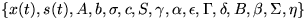is simply the product of the independent pdfs.Next: Diagonal Gaussian ensemble Up: Ensemble Learning for Independent Previous: Model selection
Harri Lappalainen
7/10/1998